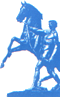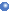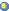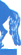RussianContactsAbout PDMI AdministrationLaboratoriesStaffSeminarsConferencesActivitiesLinksEuler IMIConferences IMI
St.Petersburg   Department   of   V.A.Steklov    Institute   of   Mathematics
of   the   Russian   Academy   of   SciencesLaboratories
Research
 Fundamental research in pure mathematics and mathematical models of theoretical physics: mathematical logic and the theory of algorithms algebra number theory geometry and topology mathematical analysis probability theory and mathematical statistics mathematical problems of continuum mechanics, quantum physics, geophysics and seismology
 ©  2008 - 2009   St.Petersburg Department of Steklov Institute of Mathematics RAS